day1:python学习爬虫抓取与解析:链家网案例

• 实验目的：爬取链家网的一些内容
• 工具：requests和BeautifulSoup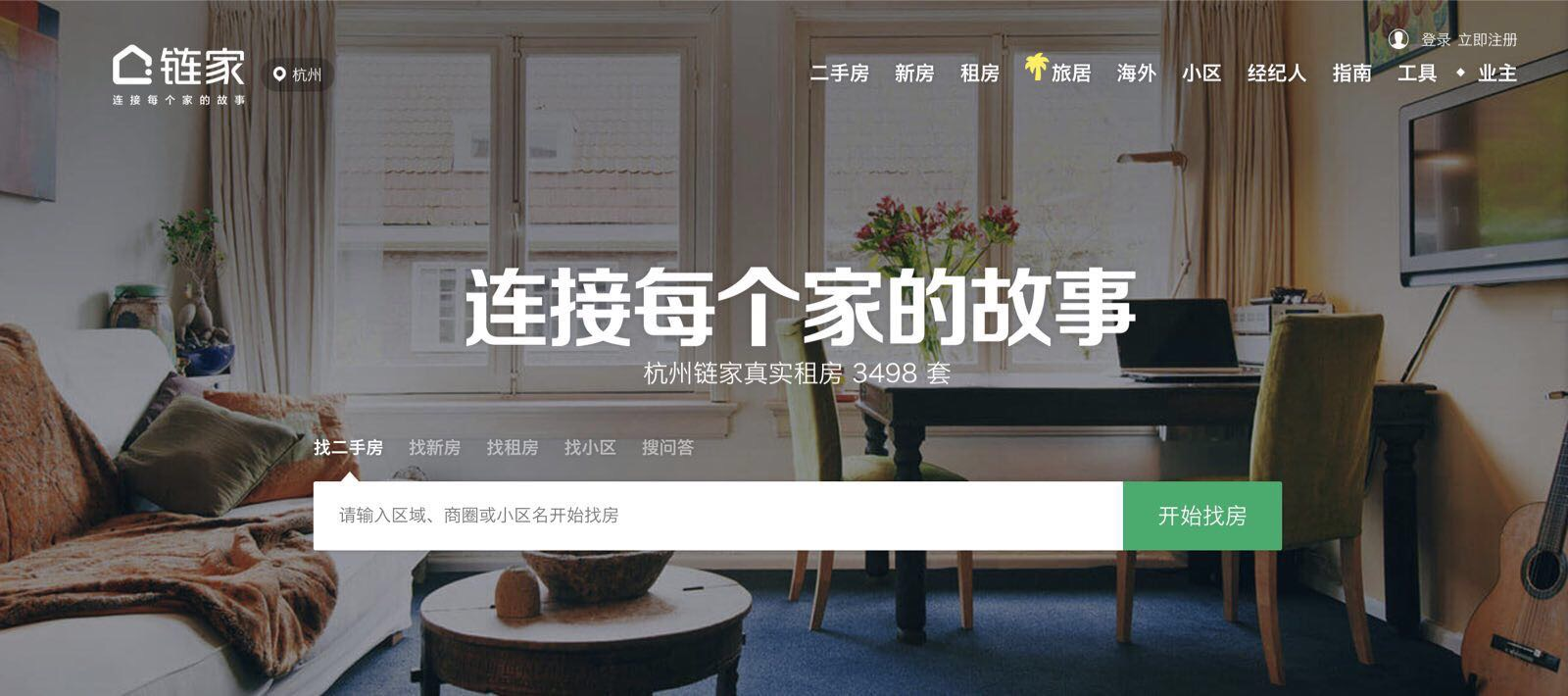1.准备工作

import requests
import time
from bs4 import BeautifulSoup

2.抓取列表页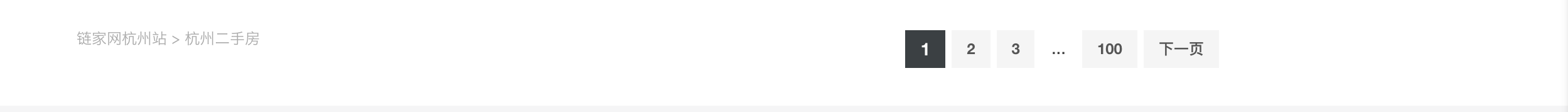URL结构为https://hz.lianjia.com/ershoufang/pg2/ 其中
- hz表示城市
- /ershoufang/是频道名称
- pg2是页面码。

#设置列表页URL的固定部分
url='http://bj.lianjia.com/ershoufang/'
#设置页面页的可变部分
page=('pg')

#设置请求头部信息
headers = {'User-Agent':'Mozilla/5.0 (Windows NT 6.1) AppleWebKit/537.11 (KHTML, like Gecko) Chrome/23.0.1271.64 Safari/537.11',
'Accept':'text/html;q=0.9,*/*;q=0.8',
'Accept-Charset':'ISO-8859-1,utf-8;q=0.7,*;q=0.3',
'Accept-Encoding':'gzip',
'Connection':'close',
}

#循环抓取列表页信息
for i in range(1,100):
if i == 1:
i=str(i)
a=(url+page+i+'/')
html=r.content
else:
i=str(i)
a=(url+page+i+'/')
html1=r.content
html = html + html1
#每次间隔1秒
time.sleep(1)

3.页面解析

#解析抓取的页面内容
wp=BeautifulSoup(html,'html.parser')

房源的总价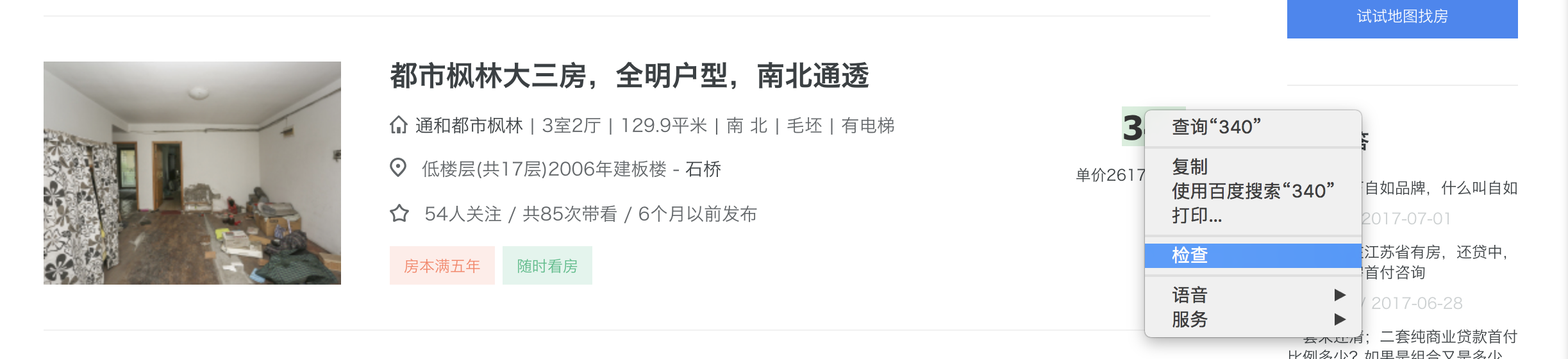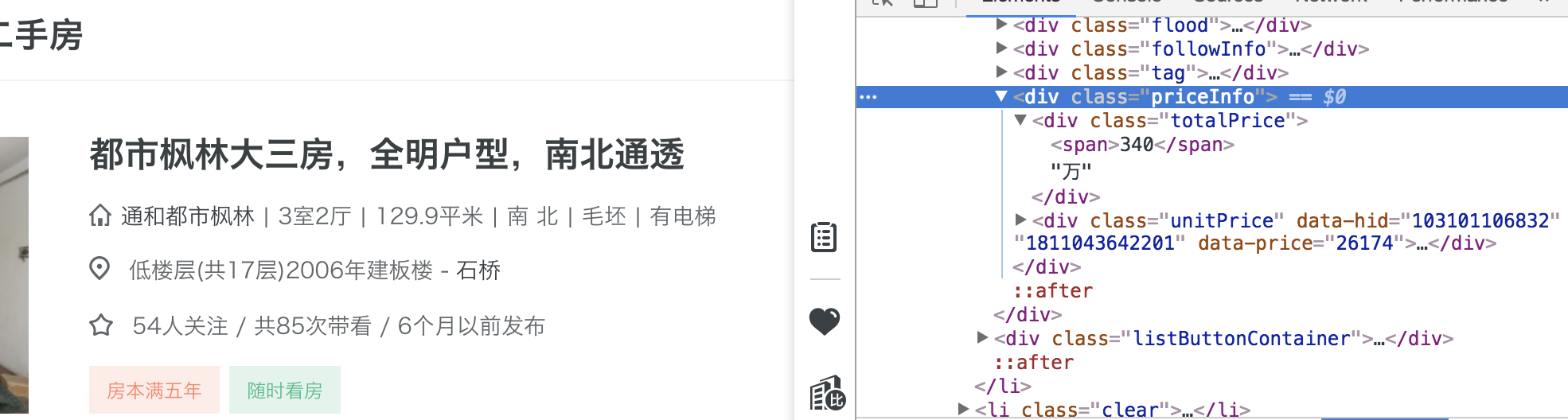#提取房源总价
price=wp.findall('div','priceInfo')
tp=[]
for a in price:
totalPrice=a.span.string
tp.append(totalPrice)

房源信息和关注度

#提取房源信息
houseInfo=lj.find_all('div',attrs={'class':'houseInfo'})
hi=[]
for b in houseInfo:
house=b.get_text()
hi.append(house)
#提取房源关注度
followInfo=lj.find_all('div',attrs={'class':'followInfo'})
fi=[]
for c in followInfo:
follow=c.get_text()
fi.append(follow)
#查看一下提取出来的数据
for item in hi:
print item

4.清洗数据

我们将之前爬取到的信息进行汇总，并导入pandas之中生成数据表。便于后面的分析。

import pandas as pd
house=pd.dataframe('TotalPrice':tp,'houseinfo':hi,'followinfo':fi)
#导入pandas库
import pandas as pd
#创建数据表
house=pd.DataFrame({'totalprice':tp,'houseinfo':hi,'followinfo':fi})
#查看数据表的内容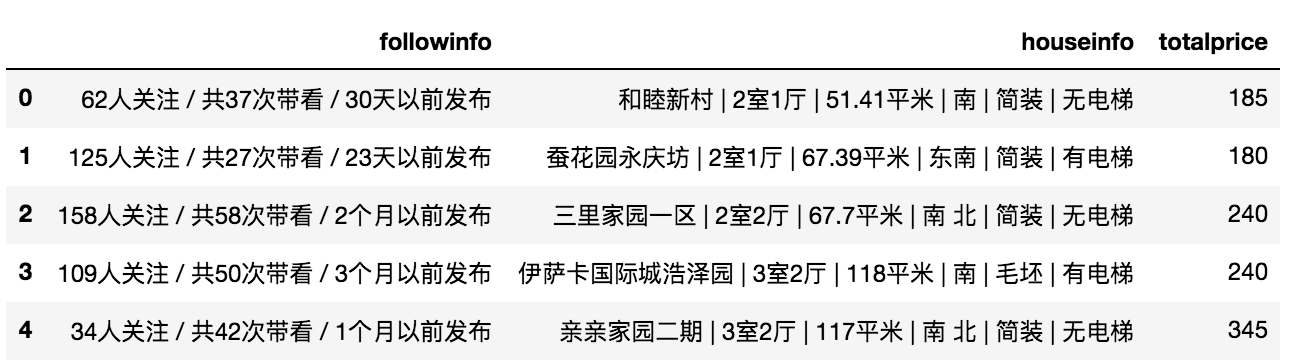#对房源信息进行分列
houseinfo_split = pd.DataFrame((x.split('|') for x in            house.houseinfo),index=house.index,columns=['小区','户型','面积','朝向','装修','电梯'])
#查看分列结果
houseinfo_split.head()
#对房源关注度进行分列
followinfo_split = pd.DataFrame((x.split('/') for x in house.followinfo),index=house.index,columns=['guanzhu','daikan','fabu'])
#将分列结果拼接回原数据表
house=pd.merge(house,houseinfo_split,right_index=True, left_index=True)
#将分列后的关注度信息拼接回原数据表
house=pd.merge(house,followinfo_split,right_index=True, left_index=True)
house.head()